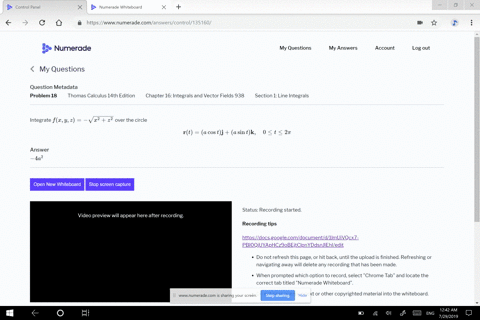🎉 The Study-to-Win Winning Ticket number has been announced! Go to your Tickets dashboard to see if you won! 🎉View Winning Ticket## Discussion

You must be signed in to discuss.

## Video Transcript

What we want to go ahead and do is we want to step through the process of how to integrate the function X y z, which is equal to X plus y Plessy all divided by, um X squared plus y squared plus c squared over. Um, the path that is represented by r of T is equal to t I plus t j plus t K and T is, um, gonna be bigger then a but smaller, then be inclusive and they are all greater than zero. Okay, so what we want to do is, first of all, we know that, um the integral is represented by the integral of the over the curve of the function eggs. Why Z integrating with respect to S and D s is equal to the magnitude of this V of tea times D t. Okay, So the first thing we needed to do is find out what the magnitude of e. Of t is and V a. T is defined be the derivative of Artie. So that's the first thing is to take the derivative and so we get I plus J plus K. And so the magnitude is the square root of those numbers in front of I, J and K or those items in front of I, J and K. So I have one squared plus one squared plus one squared, which is the square root of three and so D s is equal to the square to three TT. So this becomes integral from a to B um, of your function. And so this is going to be t plus t plus z t Sorry. Over t squared plus t squared plus C squared times the square root of three d t. So basically, what we get is on the square root of three times the integral from A to B of one over tea tt Um and that's because this becomes three t and this becomes three t squared, okay. And so we know the integral of one over T d t is equal to the natural log of the absolute value of tea. But we already know that T is restricted to positive values anyway, so t evaluated at the upper limit of B and the lower limit of a and so this is gonna be equal to, um, the square root of three times the natural log of B minus the natural log of A and using the properties of natural law, we collapse it into the natural log of be over a.